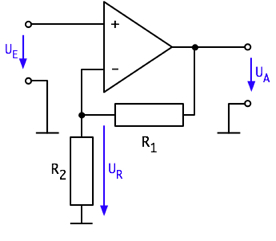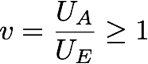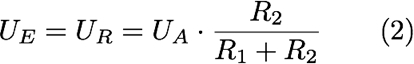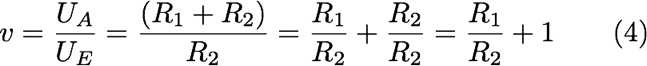## 21. January 2007 Impedance Converter And Non-inverting Amplifier

This article shows an operational amplifier (OP) that is used as an impedance converter. This ciruit will then extended to a non-inverting amplifier.

Figure 1 shows the circuit for an OP as impedance converter.Figure 1: OP as impedance converter

Impedance converters are used for weak sources to improve the output power. The name impedance converter says: a high ouput impedance or resistance of a circuit is converted to a low output impedance or resistance.

For the following observations the OP is regarded as ideal. That means the OP has an infinite high input impedance and an output impedance near Zero, a finite amplification and no offset.

An OP is a differential amplifier. That means only if there is a difference between the input signals the output will be changed.

Without additional circuit an OP behaves as follows:

If the voltage on the non-inverting input (+) is higher than the voltage on the inverting input (−) then the output voltage of the OP is positive. If the voltage on (+) is lower than on (−) then the output voltage is negative.

#### How does the circuit in figure 1 work?

Let us define that on the moment of power up there is no voltage on the inputs. So there are no differences between the inputs and the output voltage UA is therefore Zero.

If now there is a positive input voltage UE then the output voltage UA will be at first positive too, because the voltage on the inverting input is lower than UE. Because of that the voltage on the inverting input (−) raises. As long as UA is lower than UE, UA will raise further because the voltage on (+) is higher than on (−). UA will raise untill the voltage on (+) and (−) are equal. This shows that the amplification factor v of this circuit is 1.### Non-inverting amplifier

Figure 2 shows the circuit of a non-inverting amplifier.Figure 2: OP as non-inverting amplifier

For the circuit in figure 2 the definition for the ideal OP applys. The ciruit is drawn in that fashion the the relationship to the impedance converter is visible. The impedance converter is a special case of the non-inverting amplifier. The impedance converter shows the limitation of the non-inverting amplifier. The amplification factor v can never be lower than 1.#### How to calculate the amplification factor of the non-inverting amplifier?

First we look again on the moment of power on where we define that the inputs have no voltage and no difference. Because of that the output voltage UA is Zero.

When there is a positive voltage UE on the input then the output voltage UA will raise. R1 and R2 are a voltage devider for UA. The relationship R1 to R2 defines the voltage UR on the inverting input (−).The output voltage UA raises until the voltages UE and UR are equal.Converting the above equation for v=UA/UE results in the amplification factor v of this circuit:The last formulation of the equation shows immediately that in fact the amplification factor can never be lower than 1.

Consequences:

If R2 is very small the amplification will be very high. If R2 = 0 then the amplifier will work as a comparator. That means if UE is higher than 0V, then the output voltage UA will raise to its maximum. If UE is less than 0V then UA will sink to its minimum.

If R1 is very small the amplification will be very low. If R1 = 0 then the special case of the impedance converter will occur.

### Calculation examples

#### Example 1:

Given: R1 = 10kOhm; R2 = 10kOhm

Wanted: v

Solution:

According to equation (4):

v = R1/R2 + 1 = 10kOhm/10kOhm + 1 = 2

#### Example 2:

Given: v = 15; R1 = 10kOhm

Wanted: R2

Solution:

By converting of equation (4) we get:

v−1=R1/R2; R2=R1/(v−1)After applying the values:

R2=R1/(v−1)=10kΩ/(15−1)=10kΩ/14 = 714Ω

If you want to use standardized values from the E24 series you can choose between 680Ω and 750Ω. This will result in aplification factors of 15,7 with 680Ω and 14,3 with 750Ω.

#### Example 3:

Given: v=25; R2=510Ohm

Wanted: R1

Solution:

after conversion of (4) we get:

v−1=R1/R2; R1=R2*(v−1)Applying the values:

R1=R2*(v−1)=510Ω*(25−1)=12,2Ω

In this example the next value from the E24 series is 12,1kOhm. With that value the amplification factor is 24,9.